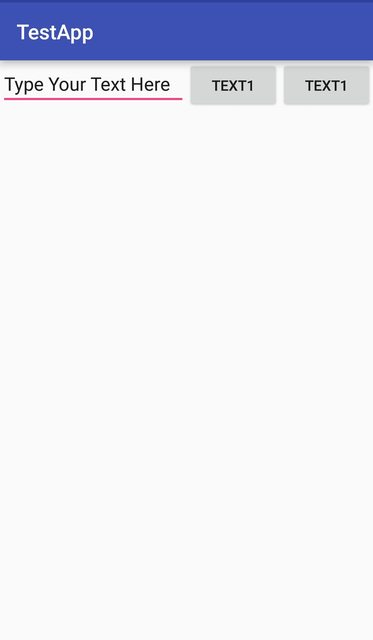# View Weight

suggest change

One of the most used attribute for LinearLayout is the weight of its child views. Weight defines how much space a view will consume compared to other views within a LinearLayout.

Weight is used when you want to give specific screen space to one component compared to other.

Key Properties:

• `weightSum` is the overall sum of weights of all child views. If you don’t specify the `weightSum`, the system will calculate the sum of all the weights on its own.
• `layout_weight` specifies the amount of space out of the total weight sum the widget will occupy.

Code:

```<?xml version="1.0" encoding="utf-8"?>
<LinearLayout xmlns:android="http://schemas.android.com/apk/res/android"
xmlns:app="http://schemas.android.com/apk/res-auto"
xmlns:tools="http://schemas.android.com/tools"
android:id="@+id/activity_main"
android:layout_width="match_parent"
android:layout_height="match_parent"
android:orientation="horizontal"
android:weightSum="4">

<EditText
android:layout_weight="2"
android:layout_width="0dp"
android:layout_height="wrap_content"

<Button
android:layout_weight="1"
android:layout_width="0dp"
android:layout_height="wrap_content"
android:text="Text1" />

<Button
android:layout_weight="1"
android:layout_width="0dp"
android:layout_height="wrap_content"
android:text="Text1" />

</LinearLayout>```

The output is:Now even if the size of the device is larger, the EditText will take 2/4 of the screen’s space. Hence the look of your app is seen consistent across all screens.

Note: Here the `layout_width` is kept `0dp` as the widget space is divided horizontally. If the widgets are to be aligned vertically `layout_height` will be set to `0dp`. This is done to increase the efficiency of the code because at runtime the system won’t attempt to calculate the width or height respectively as this is managed by the weight. If you instead used `wrap_content` the system would attempt to calculate the width/height first before applying the weight attribute which causes another calculation cycle.